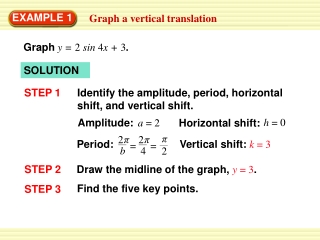DownloadDownload PresentationEXAMPLE 1

# EXAMPLE 1

Télécharger la présentation## EXAMPLE 1

- - - - - - - - - - - - - - - - - - - - - - - - - - - E N D - - - - - - - - - - - - - - - - - - - - - - - - - - -
##### Presentation Transcript

1. π π 2 2 b 4 h = 0 Amplitude: a = 2 Horizontal shift: π 2 Period: Vertical shift: k = 3 = = EXAMPLE 1 Graph a vertical translation Graphy = 2 sin 4x + 3. SOLUTION Identify the amplitude, period, horizontal shift, and vertical shift. STEP 1 STEP 2 Draw the midline of the graph, y = 3. Find the five key points. STEP 3

2. On y = k: (0, 0 + 3) = (0, 3); ( , 0 + 3) π = ( , 3); = ( , 3) ( , 0 + 3) 2 = ( , 5) Maximum: ( , 2 + 3) 3π ( , –2 + 3) 3π 8 Minimum: = ( , 1) π π π π π 8 4 8 2 8 4 EXAMPLE 1 Graph a vertical translation Draw the graph through the key points. STEP 4

3. π π 2 2 b 2 h = 3π Amplitude: a = 5 Horizontal shift: Period: Vertical shift: π k = 0 = = EXAMPLE 2 Graph a horizontal translation Graphy = 5 cos 2(x – 3π ). SOLUTION Identify the amplitude, period, horizontal shift, and vertical shift. STEP 1 STEP 2 Draw the midline of the graph. Because k = 0, the midline is the x-axis. Find the five key points. STEP 3

4. 15π 13π On y = k: = ( , 0); ( + 3π,0) 4 4 3π ( + 3π,0) 4 = ( , 0) = (3π, 5) Maximum: (0 + 3π, 5) (π+ 3π, 5) = (4π, 5) π π 7π 4 ( + 3π, –5) = ( , –5) Minimum: 2 2 EXAMPLE 2 Graph a horizontal translation Draw the graph through the key points. STEP 4

5. Ferris Wheel Suppose you are riding a Ferris wheel that turns for 180seconds. Your height h(in feet) above the ground at any time t (in seconds) can be modeled by the equation h = 85 sin (t – 10) + 90. π a. Graph your height above the ground as a function 20 of time. EXAMPLE 3 Graph a model for circular motion b. What are your maximum and minimum heights?

6. a. The amplitude is 85 and the period is = 40. The wheel turns = 4.5 times in 180 seconds, so the graph below shows 4.5 cycles. The five key points are (10, 90), (20, 175), (30, 90), (40, 5), and (50, 90). π 20 40 180 EXAMPLE 3 Graph a model for circular motion SOLUTION

7. b. Your maximum height is 90 + 85 = 175feet and your minimum height is 90 – 85 = 5feet. EXAMPLE 3 Graph a model for circular motion

8. Graph the function. y =cosx + 4. 1. for Examples 1, 2, and 3 GUIDED PRACTICE SOLUTION

9. Graph the function. y = 3 sin (x – ) 2. π 2 for Examples 1, 2, and 3 GUIDED PRACTICE SOLUTION

10. Graph the function. f(x) sin (x + π) – 1 3. for Examples 1, 2, and 3 GUIDED PRACTICE SOLUTION